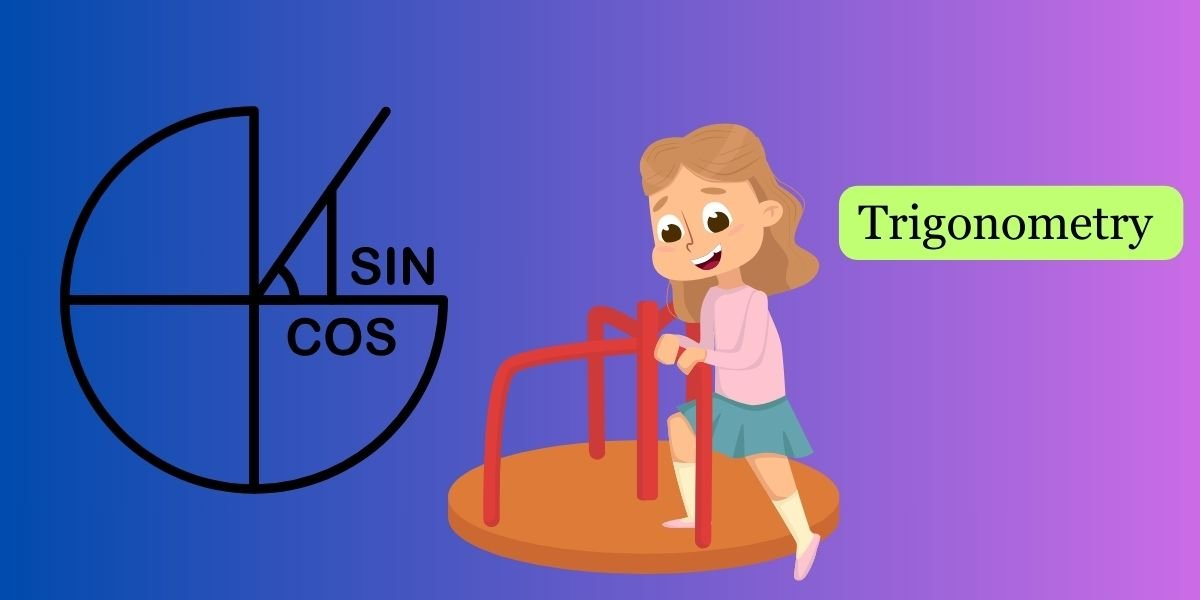Imagine you’re on a playground, and there’s a fantastic merry-go-round that spins around. This merry-go-round will help us understand some basic trigonometry concepts.

1. Circular Motion (Rotation):
• Think of the merry-go-round as a circle. When you’re on it, you’re moving around the center, just like you’d move around a clock.
• Your position on the merry-go-round can be like an angle. Imagine the center of the merry-go-round is the starting point (0 degrees), and as you move around, you measure your position in degrees.
3. Distance from the Center (Radius):
• The distance from the center of the merry-go-round to where you are is like the radius of the circle. The longer the radius, the farther you are from the center.
4. Spinning Faster or Slower (Angular Velocity):
• If the merry-go-round spins faster, you cover more degrees in less time. This is similar to the concept of angular velocity in trigonometry.

Now, let’s introduce the three main trigonometric functions using our merry-go-round:

1. Sine (Up and Down Motion):
• Imagine going up and down on the merry-go-round as it spins. The height you reach at any point can be related to the sine function. As you move up, the sine value increases.
2. Cosine (Circular Motion):
• Your horizontal motion around the merry-go-round can be related to the cosine function. It tells you where you are in terms of left and right movement. When you’re at the extreme left or right, the cosine value is 0.
3. Tangent (Slope or Steepness):
• Think of the slope or steepness of the merry-go-round as you move around. This can be related to the tangent function. As you go steeper, the tangent value increases.

So, in a nutshell, trigonometry on the merry-go-round helps us understand how your position (angle) relates to your height (sine), your horizontal position (cosine), and the slope or steepness (tangent). It’s like a mathematical adventure on a spinning playground!